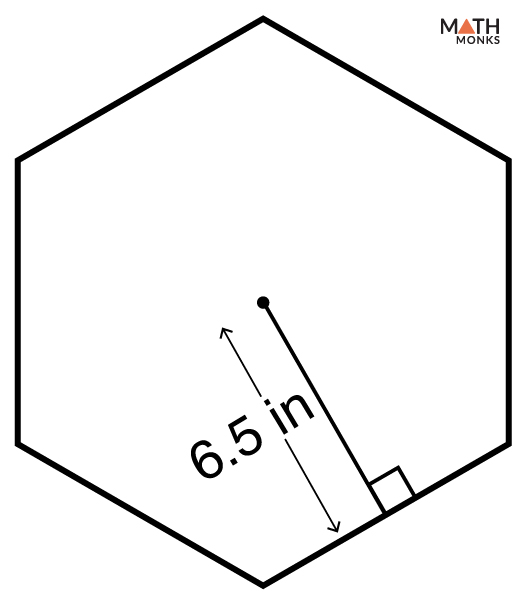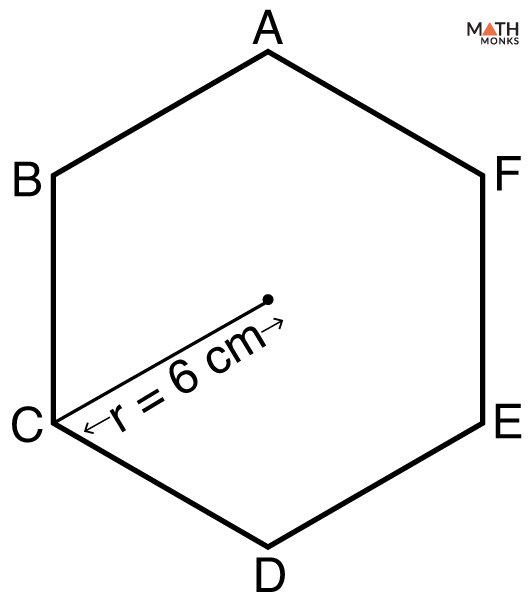# Area of Hexagon

The area of a hexagon is the total amount of space that is enclosed by all its six sides.

Since a hexagon is a two-dimensional shape, its area also lies in a two-dimensional plane. The unit of area is given in square units such as sq. m, sq. cm, sq. in or sq. ft.

## Formulas

### With Side Length

The basic formula to find the area of a regular hexagon is given below:

Let us solve some examples to understand the concept better.

Find the area of a hexagon with side length 16 cm.

Solution:

As we know,
Area (A) = ${\dfrac{3\sqrt{3}}{2}s^{2}}$ , here s = 16 cm
= ${\dfrac{3\sqrt{3}}{2}16^{2}}$
≈ 665.11 sq. cm

### With Apothem

The formula to find the area of a hexagon with apothem is given below:

Let us solve an example to understand the concept better.

Finding the area of a hexagon when APOTHEM and PERIMETER are knownFind the area of a hexagon with a perimeter of 18 in and an apothem of 6.5 in.

Solution:

As we know,
Area (A) = 1/2 × P × a, here P = 18 in, a = 6.5 in
= 1/2 × 18 × 6.5
= 58.5 sq. in

The radius of a regular hexagon is equal to its side length. Therefore the formula to find the area of a hexagon with radius is given below:Find the area of a hexagon ABCDEF with radius 6 cm.